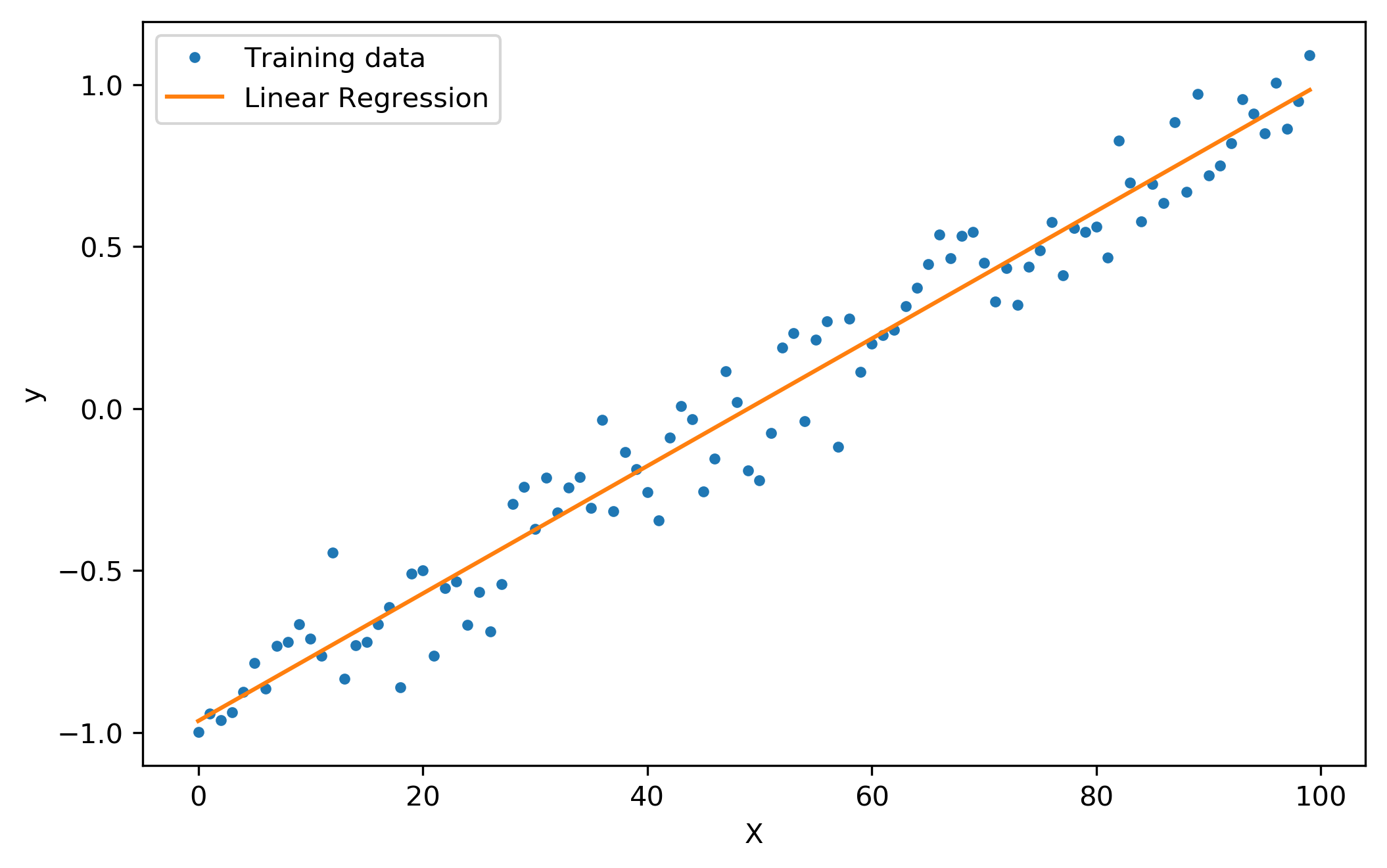# 3. Linear Regression¶

Now that we have a framework for making decisions, we can finally create our first real machine learning model. Though relatively simple, linear regression forms the basis of many more complicated models. Linear regression is in fact a very deep topic that contains lots of nuance. After defining the problem, we’ll slowly scale up to more and more complex models based on linear regression.Fig. 3.1 A depiction of linear regression.

Here’s a brief outline of what we’ll do in this section:

1. Formally introduce the linear regression setting and the assumptions we make.

2. Derive the solution to linear regression in one dimension.

3. Extend the single dimensional derivation to multiple dimensions by introducing concepts from linear algebra.

4. Explain how we can use linear regression for problems that are “non-linear”.

5. Derive some variants of linear regression.